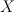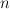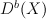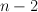## L. Katzarkov: “Gaps, Spectra, and Applications”

Here are my notes from Katzarkov’s talk in Bonn:

Katzarkov_Bonn_Sep_2010

Summary:

• Clemens-Griffiths showed that the 3-dimensional cubic is not rational by showing that its intermediate Jacobian is not the Jacobian of a curve; we suggest analogs of this.
• Homological Mirror Symmetry and the perverse sheaf of vanishing cycles
• detecting rationality via monodromy properties on the LG mirror (Gross-Katzarkov, Pryzalkowski, Golyshev)
• spectra of triangulated categories; examples
• Theorem:$X$ is rational of dimension$n$ implies that the spectrum of$D^b(X)$ has no gaps of size greaeter than$n-2$
• the outlook for 4-dimensional cubics